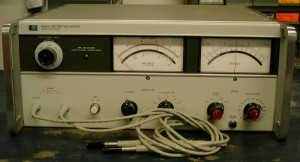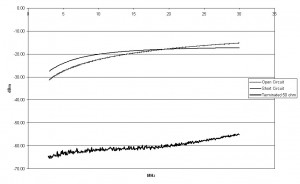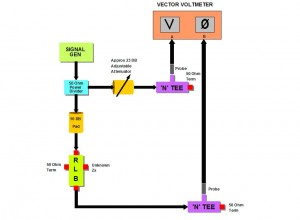Measuring Complex Impedances using the HP8405A Vector Voltmeter and a Return Loss Bridge

Measuring complex impedances above 1000Ω is usually not possible with the average Antenna analyser however using a return loss bridge and a Vector Voltmeter this becomes possible. With a return loss bridge the amplitude and phase reading of the Vector Voltmeter represent the magnitude and phase of the reflection coefficient; if we plot this on a Smith chart we can directly read the complex impedance.My first experiments were conducted with a RLB as described by Jack Priedigkeit W6ZGN in the October 1981 edition of QST this bridge was built using 51ohm chip resistors and a simple balun constructed with 30 Bifilar turns of #30 enamelled wire on a stack of four T44-1 toroidal cores. This bridge produced good performance for HF with directivity of 40dB from 40 metres to 10 metres (reflection coefficient of 0.01 or VSWR of 1.02:1) and quite usable performance down at 80 metres. (See Trace1)The only drawback with this bridge is that I get a 180 phase reversal due to the Balun so the Vector voltmeter must be zeroed at 180 degrees, then 180 degrees subtracted from the final reading. I then tried a slightly modified version of the Bridge described by Paul VK3DIP in the WIA Amateur Radio Magazine. In my unit I used 9 binocular cores and mounted the completed bridge in a box manufactured by soldering pieces of PCB together, I used N connectors instead of BNC’s and used single 51ohm chip resistors instead of two parallel 100 ohm chip resistors, this bridge produced a much greater usable frequency range however slightly less overall directivity performance, 35dB at 6 metres and 30dB at 2 metres; still a very good result as 30dB is only a return loss coefficient of 0.032 or a VSWR of 1.06:1. (See trace2)The possible reason for the diverging open and short circuit results obtained with the second bridge is possibly due to the poor quality of my short circuit constructed with a bit of wire soldered to short out a BNC connector. The traces were done using 50ohm SMA microwave terminations with a rated VSWR of 1.15:1 at 14GHz and plotted with an Agilent E4408B spectrum analyser and tracking generator, input to the bridge was 0dBm. The second RLB did not give the 180 degree phase shift of the first bridge. The results I obtained were not as good as quoted by VK3DIP however for real world matching solutions from 80 metres to 2 metres I am very happy with my results. The other change to Paul’s design was my use of semi rigid or hardline coax for the manufacture of the balun.
The Setup: (See Fig 1)The output of a signal generator is fed through a 50 ohm power divider via an adjustable attenuator to the reference A channel of the Vector Voltmeter, the second output of the power divider is fed via a 10dB pad to the input of the RLB the output of the bridge is fed via a terminated tee connector to the B channel of the Vector voltmeter.
The 50 ohm power divider is made of a resistive Y network mounted in a diecast box, each arm of the Y is made from two parallel 33 ohm Chip resistors each arm of the Y should actually be 16.66ohms however 16.5 seems to give good enough results.
The 10dB pad is used to limit the effect of varying impedances on the test output of the bridge.
The Method: Apply a signal from the generator with the bridge Zx output unterminated adjust the variable attenuator to match the A channel level to the same reading as that of the B channel (in my set-up this was about 18dB) this will vary with frequency, I also used the 10 mV range on the voltmeter scale to simplify the return loss coefficient readings. Adjust the phase control to zero the phase reading of the vector voltmeter. Now connect the unknown and plot the results directly on a Smith chart using the reflection coefficient scale at the side of the chart and the degree scale around the circumference of the chart. The plot obtained on the Smith chart is normalised so for instance if we plotted an impedance of 1.2 –j0.6 the actual impedance for a 50 ohm system would be 60 –j30 ohms. To check that I was getting valid plots I terminated the bridge with a short circuit and got a purely resistive result of about 1 ohm, I then terminated the bridge with a 75 ohm chip resistor and sure enough it plotted right on the money I then used 100 ohms and again bingo it plotted just where it should. To check the phase readings I cut a piece of coax calculated to be 0.125 wavelengths remembering to take the velocity factor into account this I measured open and short circuit and sure enough I obtained my 90 degrees both positive and negative. This satisfied me that the readings I was obtaining were actually valid.
The Matching solution: Having measured the complex impedance we are now in a position to be able to calculate a matching solution. I do not plan to go into this process here however the hard work of plotting the complex impedance is done and there are numerous tools available including on line to help calculate your matching solution.